# Machine Learning Automation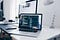Photo by Christopher Gower on Unsplash

“Just because you can automate something, it doesn’t follow that it should be automated.”

What we will be covering in this article

• Need of Automation

if you’re curious that how the final product will look like. Then have a look on my these two projects

1) Machine Learning/Data Science automation on the web

2) Machine Learning Web App (IRIS Classification)

# Now let’s start

Need Of Automation:

Automated machine learning helps enterprise users to quickly adopt machine learning solutions by automating much of the modeling activities required to build and deploy machine learning models, enabling the data scientists of a company to concentrate on more complicated issues.

Automation simplifies human activities and actually reduces operational costs in the long run. Also, a business of any size and nature can automate its processes and improve performance significantly.

1. Cost-Effectiveness

2. Time-Saving

3. Enhanced Workflow Efficiencies

4. Accuracy and Consistency in Operations

5. Reduced Employee Turnover

Can we really make a fully automated Machine Learning System?

The answer is “NO”

But

Machine learning can be automated when it involves the same activity again and again. However, the fundamental nature of machine learning deals with the opposite: variable conditions. In this regard, machine learning needs to be able to function independently and with different solutions to match different demands.

Automated machine learning changes that, making it easier to build and use machine learning models in the real world by running systematic processes on raw data and selecting models that pull the most relevant information from the data — what is often referred to as “the signal in the noise.” Automated machine learning incorporates machine learning best practices from top-ranked data scientists to make data science more accessible across the organization.

How are we going to build:

We’re going to use “Streamlit” for making it on the web and, using “Pandas” we’re going to interact with the data file and “Matplotlib” and “Seaborn” for data exploration and, now the best part we are going to use the “SweetViz” library focuses on exploring the data with the help of beautiful and high-density visualizations…

Streamlit:

Streamlit is an open-source Python library that makes it easy to create and share beautiful, custom web apps for machine learning and data science. In just a few minutes you can build and deploy powerful data apps

Pandas:

Pandas is a fast, powerful, flexible, and easy to use open-source data analysis and manipulation tool, build on top of the Python Programming Language…

Matplotlib and Seaborn:

Matplotlib is mainly deployed for basic plotting. Visualization using Matplotlib generally consists of bars, pies, lines, scatter plots, and so on.

Seaborn, on the other hand, provides a variety of visualization patterns. It uses fewer syntax and has easily interesting default themes…

SweetViz:

Sweetviz is a python library that focuses on exploring the data with the help of beautiful and high-density visualizations. It not only automates the EDA but is also used for comparing datasets and drawing inferences from them. Here we will analyze the same dataset as we used for pandas profiling.

Building an AutoML System:

`## Importing Necessary Librariesimport streamlit as stimport streamlit.components.v1 as componentsst.set_option(‘deprecation.showPyplotGlobalUse’, False)import codecsimport pandas as pd import numpy as np import matplotlib.pyplot as pltimport seaborn as sns`

Making simple Streamlit app

`import streamlit as stdef main(): st.title("Machine Learning Automation")## Eveything else inside this blockif __name__ == "__main__":    main()`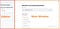Automation web app

Basically, the app has 2 main components

1. Sidebar Section

Let build these two components

Building Sidebar Section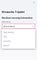Sidebar

As you can see in the image we have four option to choose from

Data Analysis, EDA, SweetViz, and optional About section

`## Side barst.sidebar.title(“Himanshu Tripathi”)st.sidebar.header(“Machine Learning Automation”)activites = [‘Data Analysis’,’EDA’,’SweetViz’,’ABOUT’] choice = st.sidebar.selectbox(“Select Actvity”, activites)`

Building drag and drop logic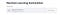Drag and drop
`data = st.file_uploader(“Upload Dataset”, type=[‘csv’,’txt’,]) df = pd.DataFrame()if data is not None: df = pd.read_csv(data) st.success(“Data File Uploaded Successfully”)`

Building our first screen which is the data analysis screen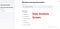Data Analytics Screen
`if choice == ‘Data Analysis’: st.subheader(“Data Analysis”) # Data Show if st.checkbox(“Show Data”):     select_ = st.radio(“HEAD OR TAIL”,(‘All’,’HEAD’,’TAIL’))     if select_ == ‘All’:         st.dataframe(df)     elif select_ == ‘HEAD’:         st.dataframe(df.head())     elif select_ == ‘TAIL’:         st.dataframe(df.tail())      # Columns    if st.checkbox(“Show Columns”):        select_ = st.radio(“Select Columns”,(‘All Columns’,’Specific Column’))    if select_ == “All Columns”:        st.write(df.columns)    if select_ == “Specific Column”:       col_spe = st.multiselect(“Select Columns To Show”,df.columns)       st.write(df[col_spe])    # Show Dimension    if st.checkbox(“Show Dimension”):       select_ = st.radio(‘Select Dimension’,     (‘All’,’Row’,’Column’))   if select_ == “All”:       st.write(df.shape)   elif select_ == “Row”:       st.write(df.shape)   elif select_ == “Column”:       st.write(df.shape)     # Summary of dataset   if st.checkbox(“Summary of Data Set”):      st.write(df.describe())    # Value Counts   if st.checkbox(“Value Count”):      select_ = st.multiselect(“Select values”,df.columns.tolist())      st.write(df[select_].count())    # Show data Type   if st.checkbox(“Show Data Type”):       select_ = st.radio(“Select “,(‘All Columns’,’Specific Column’))   if select_ == “All Columns”:       st.write(df.dtypes)   elif select_ == “Specific Column”:       s = st.multiselect(“Select value”,df.columns.tolist())       st.write(df[s].dtypes)     # Check for Null Values   if st.checkbox(“Check For Null Values”):        st.write(df.isnull().sum())`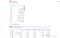Data Exploration Working

EDA (Exploratory Data Analysis) Screen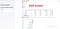EDA Screen
`#Data Visualizationelif choice == 'EDA':  st.subheader("Data Visualization")  if st.checkbox("Show Data"):   select_ = st.radio("HEAD OR TAIL",('All','HEAD','TAIL'))   if select_ == 'All':    st.dataframe(df)   elif select_ == 'HEAD':    st.dataframe(df.head())   elif select_ == 'TAIL':    st.dataframe(df.tail())  # Show Columns Names  if st.checkbox("Columns Names"):   st.write(df.columns)  # Check for null values in the form of HeatMap  if st.checkbox("Show Null Values in Heatmap"):   st.write(sns.heatmap(df.isnull()))   st.pyplot()# Quick Analysis  if st.checkbox("Quick Analysis"):   select_ = st.radio("Select Type for Quick Analysis",('Count Plot','Box Plot','Bar Plot for Specific Columns','lmplot','Scatter Plot','Correlation Heatmap','Histogram','Joint Distribution Plot'))   if select_ == "Count Plot":    st.write(df.dtypes)    s = st.text_input('Enter Column Name')    try:     if s != " ":      st.write(sns.countplot(df[s]))      st.pyplot()    except Exception as e:     st.error(e)   elif select_ == 'lmplot':    st.write(df.dtypes)    x = st.text_input('Enter X Value')    y = st.text_input("Enter Y Value")    try:     if x != " " and y != " ":      st.write(x,y)      st.write(sns.lmplot(x,y,data=df))      st.pyplot()    except Exception as e:     st.error(e)elif select_ == 'Scatter Plot':    st.write(df.dtypes)    x = st.text_input('Enter X Value')    y = st.text_input("Enter Y Value")    try:     if x != " " and y != " ":      st.write(x,y)      st.write(sns.scatterplot(x,y,data=df))      st.pyplot()    except Exception as e:     st.error(e)       elif select_ == 'Box Plot':    st.write(sns.boxplot(data=df))    st.pyplot()elif select_ == "Bar Plot for Specific Columns":    x = st.multiselect('Select Value',df.columns)    try:     if x != " ":      st.write(sns.barplot(data=df[x]))      st.pyplot()    except Exception as e:     st.error(e)elif select_ == "Correlation Heatmap":    st.write(sns.heatmap(df.corr()))    st.pyplot()elif select_ == "Histogram":    x = st.multiselect('Select Numerical Variables',df.columns)    try:     if x != " ":      st.write(sns.distplot(df[x]))      st.pyplot()    except Exception as e:     st.error(e)elif select_ == "Joint Distribution Plot":    st.write(df.dtypes)    x = st.text_input('Enter X Value')    y = st.text_input("Enter Y Value")    try:     if x != " " and y != " ":      st.write(x,y)      st.write(sns.jointplot(x,y,data=df))      st.pyplot()    except Exception as e:     st.error(e)`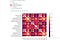Pair Plot and Correlation Heatmap

SweetViz Visualization Screen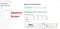Sweetviz Screen
`elif choice == 'SweetViz':  st.subheader("Automated EDA with Sweetviz")  # data_file = st.file_uploader("Upload CSV",type=['csv'])  # if data_file is not None:  # df = pd.read_csv(data)  st.dataframe(df.head())  if st.button("Generate Sweetviz Report"):   # Normal Workflow   with st.spinner("Just wait a second.. Making Something good for you... "):    report = sv.analyze(df)    report.show_html(open_browser = False)display_sweetviz("SWEETVIZ_REPORT.html")`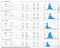SweetViz in action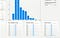More about the sweetviz

Complete Code

`import streamlit as stimport streamlit.components.v1 as componentsst.set_option('deprecation.showPyplotGlobalUse', False)import codecsimport pandas as pd import numpy as np import matplotlib.pyplot as pltimport seaborn as snsfrom sklearn.model_selection import train_test_splitfrom sklearn.preprocessing import StandardScalerfrom sklearn.metrics import confusion_matrix, accuracy_scoreimport tensorflow as tf# making custom componentimport sweetviz as svdef display_sweetviz(report_file, width=1000, height=800): report_file = codecs.open(report_file,'r') page = report_file.read() components.html(page, width=width, height=height, scrolling=True)def main(): st.title("Machine Learning Automation") st.sidebar.title("Himanshu Tripathi") st.sidebar.header("Machine Learning Automation")activites = ['Data Analysis','EDA','SweetViz','ABOUT'] choice = st.sidebar.selectbox("Select Actvity", activites)data = st.file_uploader("Upload Dataset", type=['csv','txt',])  df = pd.DataFrame() if data is not None:  df = pd.read_csv(data)  st.success("Data File Uploaded Successfully")if choice == 'Data Analysis':   st.subheader("Data Analysis")   # Data Show   if st.checkbox("Show Data"):    select_ = st.radio("HEAD OR TAIL",('All','HEAD','TAIL'))    if select_ == 'All':     st.dataframe(df)    elif select_ == 'HEAD':     st.dataframe(df.head())    elif select_ == 'TAIL':     st.dataframe(df.tail())   # Columns   if st.checkbox("Show Columns"):    select_ = st.radio("Select Columns",('All Columns','Specific Column'))    if select_ == "All Columns":     st.write(df.columns)    if select_ == "Specific Column":     col_spe = st.multiselect("Select Columns To Show",df.columns)     st.write(df[col_spe])# Show Dimension   if st.checkbox("Show Dimension"):    select_ = st.radio('Select Dimension',('All','Row','Column'))    if select_ == "All":     st.write(df.shape)    elif select_ == "Row":     st.write(df.shape)    elif select_ == "Column":     st.write(df.shape)# Summary of dataset   if st.checkbox("Summary of Data Set"):    st.write(df.describe())# Value Counts   if st.checkbox("Value Count"):    select_ = st.multiselect("Select values",df.columns.tolist())    st.write(df[select_].count())# Show data Type   if st.checkbox("Show Data Type"):    select_ = st.radio("Select ",('All Columns','Specific Column'))    if select_ == "All Columns":     st.write(df.dtypes)    elif select_ == "Specific Column":     s = st.multiselect("Select value",df.columns.tolist())     st.write(df[s].dtypes)# Check for Null Values   if st.checkbox("Check For Null Values"):    st.write(df.isnull().sum())#Data Visualizationelif choice == 'EDA':  st.subheader("Data Visualization")  if st.checkbox("Show Data"):   select_ = st.radio("HEAD OR TAIL",('All','HEAD','TAIL'))   if select_ == 'All':    st.dataframe(df)   elif select_ == 'HEAD':    st.dataframe(df.head())   elif select_ == 'TAIL':    st.dataframe(df.tail())  # Show Columns Names  if st.checkbox("Columns Names"):   st.write(df.columns)  # Check for null values in the form of HeatMap  if st.checkbox("Show Null Values in Heatmap"):   st.write(sns.heatmap(df.isnull()))   st.pyplot()# Quick Analysis  if st.checkbox("Quick Analysis"):   select_ = st.radio("Select Type for Quick Analysis",('Count Plot','Box Plot','Bar Plot for Specific Columns','lmplot','Scatter Plot','Correlation Heatmap','Histogram','Joint Distribution Plot'))   if select_ == "Count Plot":    st.write(df.dtypes)    s = st.text_input('Enter Column Name')    try:     if s != " ":      st.write(sns.countplot(df[s]))      st.pyplot()    except Exception as e:     st.error(e)   elif select_ == 'lmplot':    st.write(df.dtypes)    x = st.text_input('Enter X Value')    y = st.text_input("Enter Y Value")    try:     if x != " " and y != " ":      st.write(x,y)      st.write(sns.lmplot(x,y,data=df))      st.pyplot()    except Exception as e:     st.error(e)elif select_ == 'Scatter Plot':    st.write(df.dtypes)    x = st.text_input('Enter X Value')    y = st.text_input("Enter Y Value")    try:     if x != " " and y != " ":      st.write(x,y)      st.write(sns.scatterplot(x,y,data=df))      st.pyplot()    except Exception as e:     st.error(e)       elif select_ == 'Box Plot':    st.write(sns.boxplot(data=df))    st.pyplot()elif select_ == "Bar Plot for Specific Columns":    x = st.multiselect('Select Value',df.columns)    try:     if x != " ":      st.write(sns.barplot(data=df[x]))      st.pyplot()    except Exception as e:     st.error(e)elif select_ == "Correlation Heatmap":    st.write(sns.heatmap(df.corr()))    st.pyplot()elif select_ == "Histogram":    x = st.multiselect('Select Numerical Variables',df.columns)    try:     if x != " ":      st.write(sns.distplot(df[x]))      st.pyplot()    except Exception as e:     st.error(e)elif select_ == "Joint Distribution Plot":    st.write(df.dtypes)    x = st.text_input('Enter X Value')    y = st.text_input("Enter Y Value")    try:     if x != " " and y != " ":      st.write(x,y)      st.write(sns.jointplot(x,y,data=df))      st.pyplot()    except Exception as e:     st.error(e)# st.write(sns.countplot(df[str(s)]))    # st.pyplot()# Sweet viz elif choice == 'SweetViz':  st.subheader("Automated EDA with Sweetviz")  # data_file = st.file_uploader("Upload CSV",type=['csv'])  # if data_file is not None:  # df = pd.read_csv(data)  st.dataframe(df.head())  if st.button("Generate Sweetviz Report"):   # Normal Workflow   with st.spinner("Just wait a second.. Making Something good for you... "):    report = sv.analyze(df)    report.show_html(open_browser = False)display_sweetviz("SWEETVIZ_REPORT.html")elif choice == 'ABOUT':  st.subheader("About Me")if __name__ == "__main__": main()`

“Automation isn’t a silver bullet, and it won’t fix your broken processes for you.”

Check out more interesting Machine Learning, Deep Learning, Data Science Projects on my YouTube 👉 :- YouTube ( 👍)

That’s it for now 👏👏. See you in the next Article.

Check out my previous articles:

If you found this article interesting, helpful and if you learn something from this article, please clap(👏👏) and leave feedback.

Thanks for reading!

## Nerd For Tech

From Confusion to Clarification

Written by

## Himanshu Tripathi

Former Natural Language Processing Intern at DRDO || Machine Learning || Deep Learning || Data Science || Web Developer || Android Developer (UI) ||

Written by

## Himanshu Tripathi

Former Natural Language Processing Intern at DRDO || Machine Learning || Deep Learning || Data Science || Web Developer || Android Developer (UI) ||